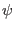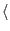Next: Superposition and Eigenstates Up: The Quantum Computer Previous: The Classical Bit   Contents

## State Vectors and Dirac Notation

We wish to know exactly how the behavior of the spin-1/2 particle, our qubit, differs from a that of a classical bit. Recall that a classical bit can store either a 1 or a 0, and when measured the value observed will always be the value stored. Quantum physics states that when we measure the spin-1/2 particles state we will determine that it is in the +1/2 state, or the spin -1/2 state. In this manner our qubit is not different from a classical bit, for it can be measured to be in the +1/2, or 1 state, or the -1/2, or 0 state. The differences between the qubit and the bit come from what sort of information a qubit can store when it is not being measured.

According to quantum physics we may describe the state of this spin-1/2 particle by a state vector in a Hilbert Space.

A Hilbert Space is a linear, complex vector space.

In a linear vector space you may add and multiply vectors that lie within the space together and the resulting vector will still lie within that space. For example you are probably familiar with the x, y, z coordinate system where the x, y, and z axes are mutually perpendicular real number lines, which coincide at the point x = 0, y = 0, z = 0 - this is an example of a linear vector space.

In a complex vector space the lengths of the vectors within the space are described with complex numbers. Complex numbers are numbers which take the form a + i*b, where a and b are real numbers, and i is defined to be the square root of negative one. (Williams, Clearwater)

In the Hilbert Space for our state vector, which describes the state of our spin-1/2 particle, these perpendicular axes will correspond to each possible state that the system can be measured in. So our Hilbert Space for a single qubit will have two perpendicular axes, one corresponding to the spin-1/2 particle being in the +1/2 state, and the other to the particle being in the -1/2 state. These states which the vector can be measured to be are referred to as eigenstates. The vector which exists somewhere in this space which represents the state of our spin-1/2 particle is called the state vector. The projection of the state vector onto one of the axes shows the contribution of that axes' eigenstate to the whole state. This means that in general, the state of the spin-1/2 particle can be any combination of the base states. In this manner a qubit is totally unlike a bit, for a bit can exist in only the 0 or 1 state, but the qubit can exist, in principle, in any combination of the 0 and 1 state, and is only constrained to be in the 0 or 1 state when we measure the state.

Now I will introduce the standard notation for state vectors in Quantum physics. The state vector is written with the following way and called a ket vector |. Whereis a list of numbers which contain information about the projection of the state vector onto its base states. The term ket and this notation come from the physicist Paul Dirac who wanted a concise shorthand for writing formulas that occur in Quantum physics. These formulas frequently took the form of the product of a row vector with a column vector. Thus he referred to row vectors as bra vectors represented asy|. The product of a bra and a ket vector would be writteny| x, and would be referred to as a bracket. (Williams, Clearwater)

There is nothing special about this vector notation, you may think of any state vector being written as a letter with a line over it, or as a bold letter as vectors are normally denoted. If you do further reading into this area you will almost assuredly come across the bra and ket notation, which is why it is presented.Next: Superposition and Eigenstates Up: The Quantum Computer Previous: The Classical Bit   Contents
Matthew Hayward - Quantum Computing and Shor's Algorithm GitHub Repository